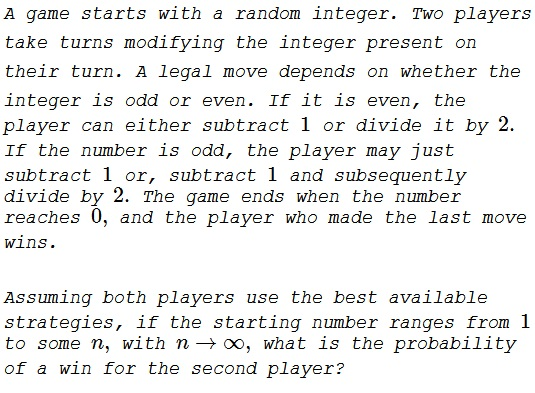# Playing with Integers and Limits

### Problem### Hint

Play the game with small starting numbers.

### Investigation and Proposition

If the starting number is $1,$ the player whose turn to make a move (i.e. the first player) wins.

If the starting number is $2,$ regardless of the move, the first player leaves $1,$ meaning that the second player wins.

If the starting number is $3,$ the first player may leave either $1$ or $2$ become the second player on the next move. A good player would leave $2$ and win the game.

Here a proposition:

If the starting integer $N$ has an even number of factors of $2$ (in particular when $N$ is odd), the first player should win. If the starting integer $N$ has an odd number of factors of $2,$ the second player should win.

### Solution

We'll prove the above statement by strong induction.

Assume it is true for any $N\le k,$ for some $k\ge 1.$ Consider $N=k+1.$ There are three cases:

Case 1: $k+1$ is odd

The first player may move to either $k$ or $k/2.$ One of $k$ or $k/2$ has an odd number of factor of $2.$ According to the proposition, this is the number the first player should live on the first move, after which he becomes the second player and wins.

Case 2: $k+1$ is even and has an odd number of factors of $2.$

$k$ is odd. And if left, the other player wins. If the first player leaves $(k+1)/2,$ which has an even number of factors $2,$ the other player still wins.

Case 3: $k+1$ is even and has an even number of factors of $2.$

The first player loses if he leaves the odd $k.$ If he leaves $(k+1)/2,$ then, according to case 2, he will win after becoming the second player.

Thus, in the cases 1 and 3, the first player should win; in the case 2, the second player wins. Thus to repeat, the second player wind if the starting number has an odd number of factors $2.$ How many such numbers are there? Let's count:

Every number in the form $2n,$ where $n$ is odd: these are numbers $2, 6, 10, \ldots.$ Every fourth integer is in this form. So the probability of falling into that group is $\displaystyle \frac{1}{4}.$

Every number in the form $8n,$ where $n$ is odd: these are numbers $8, 24, 40, \ldots.$ These numbers differ by $16=4^2.$ So every $\displaystyle \frac{1}{16^{th}}$ integer falls into that group.

We may continue in this manner, but it becomes clear that the proportion of integer having an odd number of factors $2$ is the sum of the series

$\displaystyle \frac{1}{4}+\frac{1}{4^2}+\frac{1}{4^3}+\cdots=\frac{1}{4}\cdot\frac{1}1-\frac{1}{4}}=\frac{1}{3}$

This is (more or less) the probability of a win for the second player.

### Solution 2

We claim that the starting number $n$ for the first player uniquely determines the outcome of the game. If this claim is true, then we can define a function $f$:

$f(n)= \begin{cases} 1, & \text{if player 1 wins} \\ 0, & \text{if player 2 wins.} \end{cases}$

Clearly, $f(1)=1$ and $f(2)=0$. $f(2)=0$ because if the first player starts at $2$, the only choice available is $1$ and then the second player wins by going from $1$ to $0$. We validate our claim by showing that the function gets recursively defined for all $n$ in the following way. If the possible two outcomes of the current move lead to a place where the person making the next move is guaranteed to win, then the person making the current move is guaranteed to lose. Hence,

$f(2k)= \begin{cases} 0, & \text{if}~f(2k-1)=1~\text{and}~f(k)=1 \\ 1, & \text{otherwise}; \end{cases}$

and

$f(2k+1)= \begin{cases} 0, & \text{if}~f(2k)=1~\text{and}~f(k)=1 \\ 1, & \text{otherwise}. \end{cases}$

The definition of $f(2k)$ leads to the following implication:

$f(k)=0 \Rightarrow f(2k)=1.$

Thus, the first condition in the definition of $f(2k+1)$ can never be satisfied and $f(2k+1)=1$ unconditionally. This in turn proves the converse of the preceding implication:

$f(k)=0 \iff f(2k)=1.$

Thus, from the definition of $f(2k)$, $f(4k+2)=0$ because $f(4k+1)=1$ and $f(2k+1)=1$. Thus, the only numbers left to investigate are the numbers of the form $4k$. Because multiplying by $2$ flips the function, we end up with the following tree like structure:The nodes show the form of the number and the number in the parenthesis shows the value of $f(n)$. Thus, the probability that the second player wins is the probability of getting $f(n)=0$. The required probability is

\displaystyle \begin{align} P=&\frac{1}{4}+\frac{1}{16}+\frac{1}{64}+\ldots \\ =&\sum_{m=1}^{\infty}\frac{1}{4^m} =\frac{1/4}{1-1/4}=\frac{1}{3}. \end{align}

### Acknowledgment

The problem is #68 from A Mathematical Orchard by M. I. Krusemeyer, G. T. Gilbert, L. C. Larson (MAA, 2012). Solution 2 is by Amit Itagi.